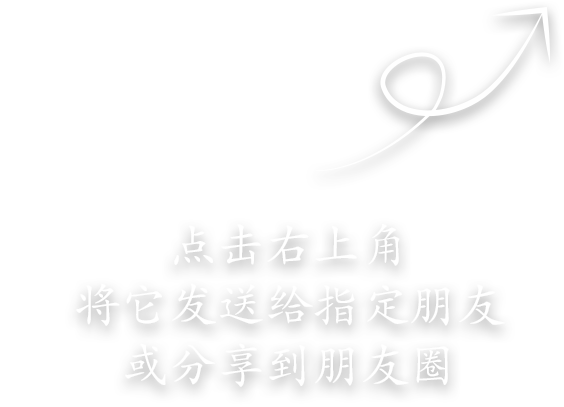• 手机版

用手机扫一扫

• 会员中心you postion

# conductance

unknown unknown 发布日期：2023-01-02 21:46:39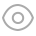68

The greater the resistance of a conductor, the worse its conductivity. On the contrary, the smaller the resistance, the better its conductivity. So the reciprocal of resistance R The reciprocal of resistance is the size of conductance, which indicates the conductivity of the conductor. Therefore, conductance is a measurement of the ability of an object or circuit to transmit current from one point to another. Its size is related to the conductivity of the object and its geometric shape and size. In electrotechnics, the ability of an object to conduct current is represented by conductance, and its symbol is represented by the capital letter "G". Therefore, the calculation formula of conductance is:

Conductivity calculation formula

The unit of conductance is "Siemens (S)" or mho (conductance unit: mho). Similarly, resistivity ρ The reciprocal of (pronounced "rou") is called conductivity, which is usually composed of Greek letters σ (Sigma), but κ (Cabo) (especially in our electrical engineering), occasionally used γ (gamma).

Resistance and conductance are indicated by the following simple symbols in the circuit:

Symbols of resistance and conductance

By the way, when the metal starts to cool down from the greenhouse, the resistivity of the metal decreases (as we all know, the resistance of many metals will change with the temperature, and the conductivity remains the same). The lower the temperature is, the smaller the resistivity will be (for most of them). Some conductors have a critical temperature. When the temperature is lower than the critical temperature, the resistance of the conductor is almost equal to 0. In this case, the conductor becomes "superconducting". In electric power engineering, the use of cryogenic technology to create conditions conducive to superconductivity will greatly reduce the size of equipment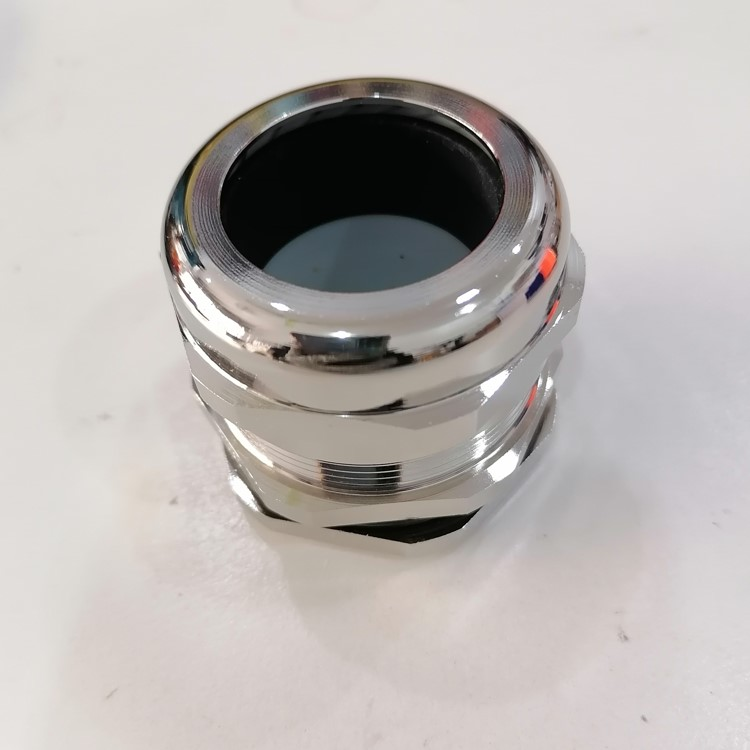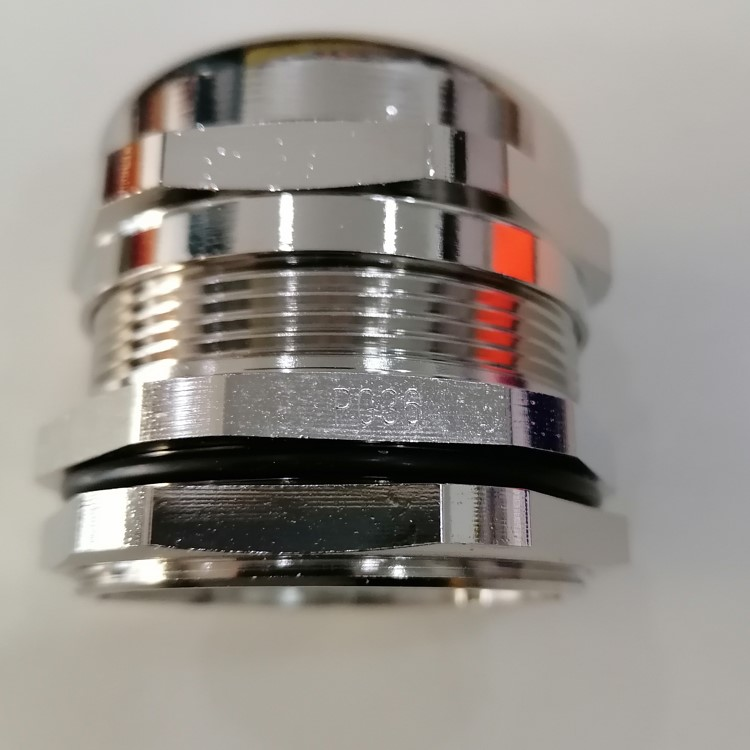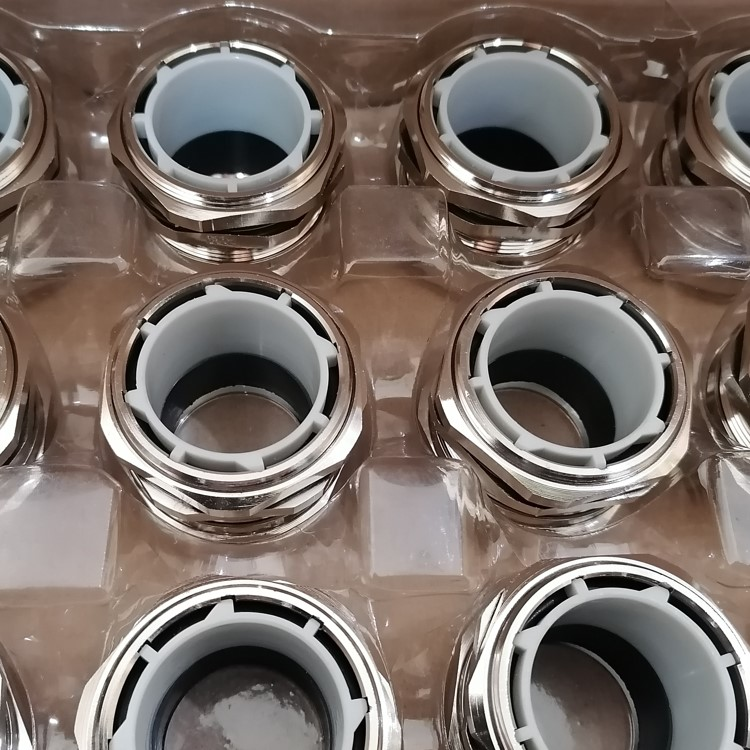Therefore

Shanghai Fakong Industrial and Trading Co.,Ltd.管理网站举报反馈网站统计